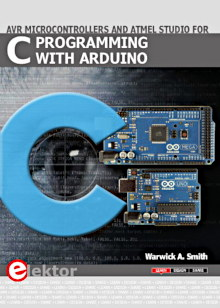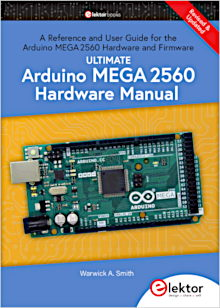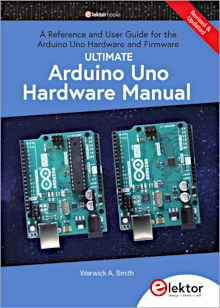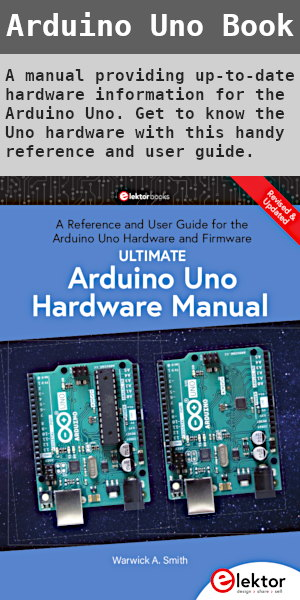Starting Electronics needs your help! Please make a donation to help cover our hosting and other costs. Click the donate button to send a donation of any amount.# Tutorial 13: VHDL Data Types and Operators

Created on: 12 February 2013

We have mainly been using the STD_LOGIC and STD_LOGIC_VECTOR data types so far in this course. The BIT_VECTOR data type was introduced in the previous tutorial.

This part of the course will look at some of the other data types that are available in VHDL as well as VHDL operators. We will then look at which VHDL operators can operate on which data types.

This is not a full list of all the data types and operators in VHDL. It also does not contain an explanation of all the operators shown, rather the purpose of this part of the course is to show you where the data types and operators that you have been using so far fit into the bigger picture of VHDL.

## VHDL Data Types

VHDL has a set of standard data types (predefined / built-in). It is also possible to have user defined data types and subtypes.

Some of the predefined data types in VHDL are: BIT, BOOLEAN and INTEGER.

The STD_LOGIC and STD_LOGIC_VECTOR data types are not built-in VHDL data types, but are defined in the standard logic 1164 package of the IEEE library. We therefore need to include this library in our VHDL code and specify that the STD_LOGIC_1164 package must be used in order to use the STD_LOGIC data type:

```library IEEE;
use IEEE.STD_LOGIC_1164.ALL;
```

We will look at only the data types used so far in the course, namely STD_LOGIC and BIT, as well as their vector forms: STD_LOGIC_VECTOR and BIT_VECTOR.

### BIT

The BIT data type can only have the value 0 or 1. When assigning a value of 0 or 1 to a BIT in VHDL code, the 0 or 1 must be enclosed in single quotes: '0' or '1'.

### BIT_VECTOR

The BIT_VECTOR data type is the vector version of the BIT type consisting of two or more bits. Each bit in a BIT_VECTOR can only have the value 0 or 1.

When assigning a value to a BIT_VECTOR, the value must be enclosed in double quotes, e.g. "1011" and the number of bits in the value must match the size of the BIT_VECTOR.

### STD_LOGIC

The STD_LOGIC data type can have the value X, 0, 1 or Z. There are other values that this data type can have, but the other values are not synthesizable – i.e. they can not be used in VHDL code that will be implemented on a CPLD or FPGA.

These values have the following meanings:

• X – unknown
• 0 – logic 0
• 1 – logic 1
• Z – high impedance (open circuit) / tristate buffer

When assigning a value to a STD_LOGIC data type, the value must be enclosed in single quotes: 'X', '0', '1' or 'Z'.

### STD_LOGIC_VECTOR

The vector version of the STD_LOGIC data type. Each bit in the set of bits that make up the vector can have the value X, 0, 1 or Z.

When assigning a value to a STD_LOGIC_VECTOR type, the value must be enclosed in double quotes, e.g. "1010", "ZZZZ" or "ZZ001". The number of bits in the value must match the size of the STD_LOGIC_VECTOR.

## VHDL Operators

Some of the VHDL operators are listed below. Not all operators can operate on all data types. Operators will be explained as they are used in this course.

### Logical Operators

NOT, AND, NAND, OR, NOR, XOR and XNOR.

### Arithmetic Operators

• - subtraction
• * multiplication
• / division
• ABS absolute value
• MOD modulus
• REM remainder
• ** exponent

### Comparison Operators

• = equal to
• /= not equal to
• < less than
• > greater than
• <= less than or equal to
• >= greater than or equal to

### Shift Operators

• sll – shift left logical
• srl – shift right logical
• sla – shift left arithmetic
• sra – shift right arithmetic
• rol – rotate left
• ror – rotate right

Books that may interest you:## VHDL Operators usable on Data Types

The following operators can be used on the data types listed.

### Logical Operators

Logical operators can operate on:

BIT and BIT_VECTOR

STD_LOGIC and STD_LOGIC_VECTOR

(also on other data types: STD_ULOGIC and STD_ULOGIC_VECTOR)

### Arithmetic Operators

The STD_LOGIC_VECTOR data type can be used in addition and subtraction operations (+ and -) if the STD_LOGIC_SIGNED or the STD_LOGIC_UNSIGNED package of the IEEE library is used.

(Otherwise the arithmetic operators can only be used with INTEGER, SIGNED and UNSIGNED data types)

### Comparison Operators

BIT and BIT_VECTOR

STD_LOGIC and STD_LOGIC_VECTOR

(also on STD_ULOGIC, STD_ULOGIC_VECTOR, INTEGER, SIGNED and UNSIGNED)

### Shift Operators

Shift operators can only be used on BIT_VECTOR data types.